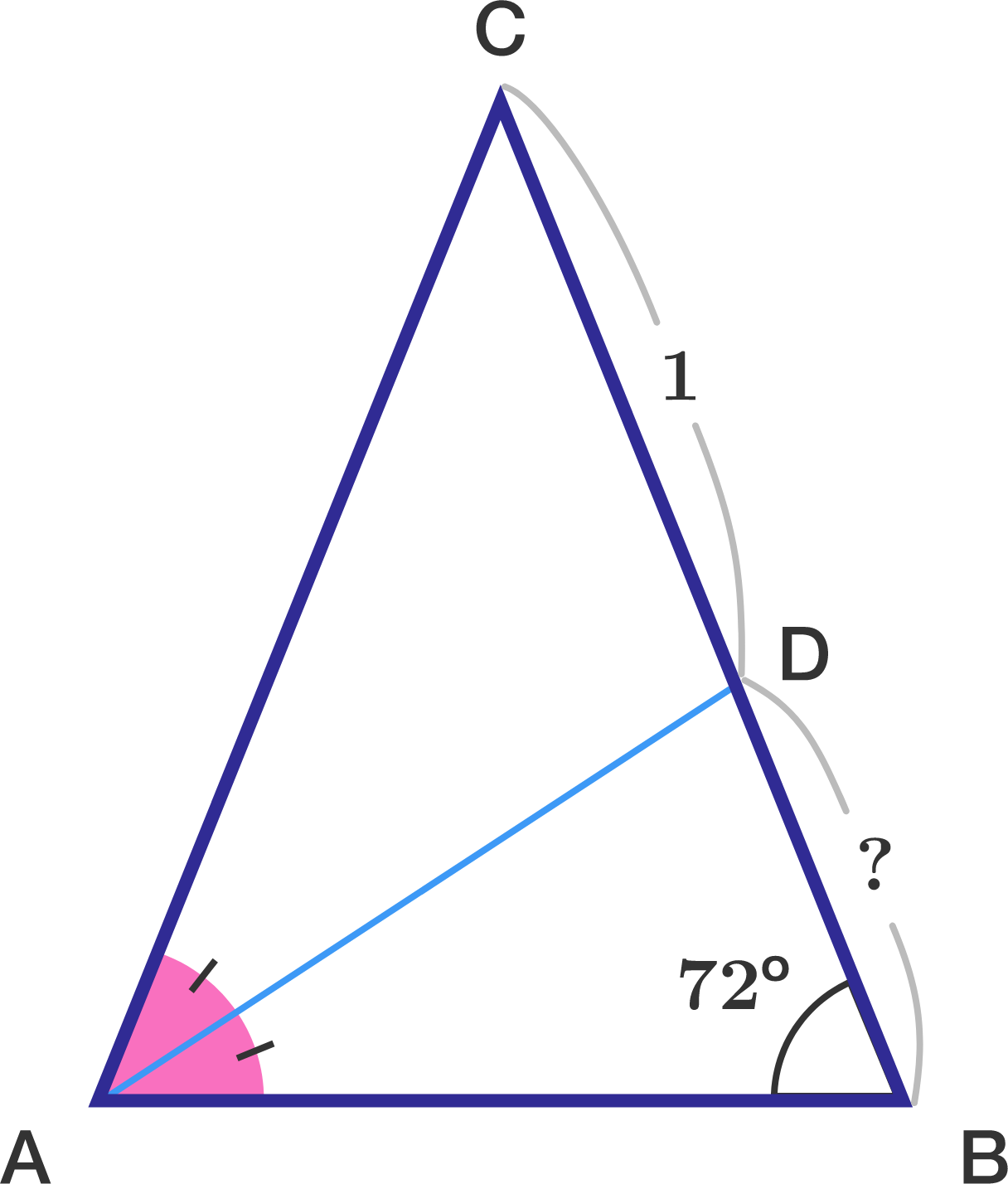# Triangle.$ABC$ is an isosceles triangle with $AC = BC$. Furthermore, $D$ is a point on $BC$ that bisects the angle at $A$.

If $\angle B = 72^\circ$ and $CD=1,$ then find length of $BD$ (upto 3 decimal places).

×